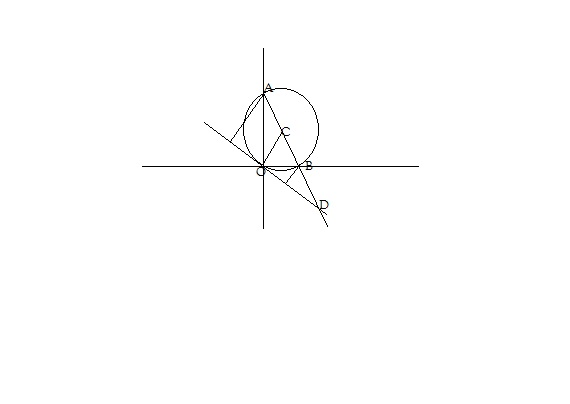# A line meets the co-ordinate axes in A and B. A circle is circumscribed about the triangle OAB. If m and n are the distances of the tangents to the circle at the origin from the points A and B respectively, the diameter of the circle is(A) m(m+n)                               (B) (m+n)(C) n(m+n)                                (D) (m+n)/2

11 years ago

Hi Sohan,

Let the line AB(extended) meet, the tangent at D (ref FigSo length of perpendicular from B = n (say it meets tangent at B')

Length of perpendicular from A = m (say it meets tangent at A')

Let DB = x, and radius of circle = r.... So AC=BC=OC=r (AB is diameter, cos at O it subtends 90 degree, angle in semicircle).

So by similar triangles:

x/(x+r) = n/r

Asnd x/(x+2r) = n/m

Solve for r, you get r = (m+n)/2

So dia = m+n.

Hence Option (B)

Best Regards,